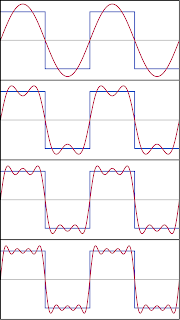## Friday, April 2, 2010

### Fourier analysis - Sines and Integrals (part 2)

In the last post, I attempted to remind the reader of the definition of a sine curve. I particularly wanted to highlight that the sine function is the mathematical representation of a wave, and since waves are representations of musical notes, a sine curve is also a mathematical representation of a musical note. Those who are familiar with music (or perhaps with my post on the guitar back in November) will be aware that different musical notes are simply waves with different frequencies. That is easily related to the sine curve by multiplying the variable by some number. For example, I've been showing plots of just the basic sine function, y = sin(x) or y = sin(θ). If, however, I decided to plot y = sin(3x), then all of a sudden the frequency of the wave would be tripled, as in this figure:It is pretty clear that during the time it takes the standard sine function (the red curve) to undergo a full oscillation, the higher frequency curve (the green curve) represented by y = sin(3x) has undergone three full oscillations. Thus, I can represent any of the musical notes using the sine function, simply by changing the multiplication factor. This is essentially what I did in the graphical representations from the November post, by just adding different functions together to produce the sounds I wanted.

We are now ready to talk about the two fundamental keys to Fourier analysis. The first is that any periodic signal can be obtained simply by adding sine curves of different frequencies. To illustrate this, I'm going to draw on everyone's friend, Wikipedia, which has a great entry on Fourier analysis and Fourier series (which does raise the question, "why am I bothering to do this when so many other people have already done it before?" but then this is my blog and I can do it again if I want to. In general, for those who are interested, Wikipedia is really good at mathematical concepts, and I use it as a reference all the time). In the Fourier series article, the unnamed Wikipedia author is exactly illustrating the point I'm making here, that any periodic function can be expressed as the sum of sine curves with different frequencies.

The first example is the square wave. This is a wave that alternates between two values, for example either 1 or -1, so that it looks like a box. The sine function is very smooth, so it may seem hard to believe that you can get a square wave from sines. But, as in the following picture, it doesn't take very many iterations before the sines do a pretty good job at imitating the square wave:A second example, featuring our favorite gimmick animation, is the the sawtooth wave. Assuming I get this to work right, the animation should show a sawtooth wave along with the sum of sines as each additional sine is added to the total. As with the square wave, the approximation gets pretty good without too many steps:

From here, it should be easy to imagine creating the shapes from my guitar post simply by adding different notes together. The second key will be the subject of the next post.